Deep Learning on Graphs For Computer Vision — CNN, RNN, and GNN

This article is based on the Paper Reading Group event at UTMIST, presented by Huan Ling, a researcher at the University of Toronto, Vector Institute, and Nvidia Research Lab. We will first attempt to define graph neural networks, and then examine a few papers that use them to some extent.

Learning on Graphs

Graphs are a fundamental data structure in computer science, but it is also a natural way to represent real-world information.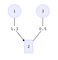A graph of 3 nodes and 2 edges

A graph is a collection of nodes and edges. A node can represent any object. Edges connect nodes to indicate some kind of relationships.

A node can store information. For example, in the graph on the left (above for mobile users), each node stores a number. It can also store a vector, a string, or anything. A node can also have labels or types. For example. we have two circular nodes and one square node.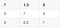The adjacency matrix for the graph above. Rows indicate starting node; Columns indicate ending node.

An edge can be directed or undirected. 1 → 2 is directed, whereas 2–3 is not. A directed edge can be used to model one way relationships such as one event happening after another, one event causing another, and one entity belonging to another. An undirected edge can model mutual relationships such as friendship, similarity, or when the direction is unclear. A graph can be equivalently represented as an Adjacency Matrix.

For this graph, 1 connects to 2 with weights 1.3, so we put it in the first row, second cell. The diagonals indicate self-connections and have different representations. In some context, it makes sense that a node can always access its own information, so we put a connected value. In other cases, a node’s information should exclusively come from its connected nodes (also called neighborhood), in which case we put a disconnected value. The representation for disconnection is also flexible. In the case that weights indicate “distance”, e.g. it takes 1.3 km to go from 1 to 2, disconnection should be represented with infinite weight. However, when we need to multiply a vector with the adjacency matrix — a method to perform updates on each node’s value based on its neighbors — it makes sense to set it to 0 so that disconnected nodes don’t influence each other.

Here are a few real-world examples modeled with graphs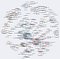A citation network for Philosophy where each node is a paper and each edge means “cite” (Image credit from Kieran Healy)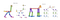A graph representation of animal skeletons, each node is a body part (image credit from Wang et al 2017)

There is a type of graph you probably see every day — a Neural Network! For notation’s sake, in this article, we will refer to a “node” in a neural network as a neuron, and a “node” in a graph representing the real-world data as actually a node. A neuron in a neural network layer may directly map to a node in the graph, but it doesn’t have to.

The definition for Graph Neural Network (GNN) is still evolving, but here we loosely define GNN as a family neural networks that take graph-structured inputs and have propagation rules designed with the graph structure in mind. That is, functions on inputs are defined in the language of nodes, edges, and neighborhoods. However, the propagation rules should not make any assumptions about the structure of the graph. Even though the inputs and computations are graph-structured, the model does not have to make a graph-structured prediction. It can be a yes/no, a sequence, or anything derivable from the graph. We will first compare traditional neural networks with GNN to see differences and similarities.

Fully Connected Neural Network

This is arguably the “hello world” neural network. Each neuron in a layer connects to every neuron in the next layer.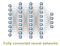A fully connected neural network with 3 hidden layers. Each neuron derives information from all neurons in the previous layer

The network adjusts weights used to propagate from one layer to the next to fit some dataset. If it deems one neuron not influential to the value of a particular neuron in the next layer, the weight will tend to zero. For a graph neural network, we can customize the connections between neurons based on real-world information about the task at hand.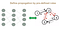The neural network (left) is an equivalent representation of the graph (right). We only connect the neurons that represent connected nodes in the graph

In this example, we connect the first neuron in layer 1 to the second neuron in layer 2 because on the graph node 1 goes to node 2. However, there is no direct connection from 1 to 3. This discourages the model from learning direct relationships between 1 and 3, but still allows the possibility for node 1 to influence node 3 in a deeper relationship through node 2 and 4. If physically this is indeed true, we spare a significant number of training iterations.

CNN

In a convolutional neural network, common for computer vision tasks, we have a moving “observation window” (convolution filter) from which the model tries to learn weights of the filter. The weights are shared for each convolution, which ensures translational equivariance, allowing the model to recognize objects wherever they show up in the image.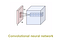A convolution of size 32x32x3 is scanned across a bigger image

In the language of graphs, we can think of CNN as a specialized graph where the convolution is a square “neighborhood” around the center node/pixel.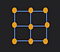For example, each convolution consists of 9 square pixels (nodes), or 8 special neighbor nodes around a center node (4 direct descendants + 4 diagonal descendants). Screenshots from the presentation (https://www.youtube.com/watch?v=WyjiBNiWx-c) Qi et al

However, we don’t always have to take neighborhoods like that. We can define neighborhoods like this: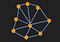An arbitrarily defined neighborhood (not the whole graph). Same source as above.

This flexibility is particularly helpful when we know physically that pixels should not be simply measured by 2D Euclidean distance. We will see this in action in the RGBD Semantic Segmentation example.

RNN

Recurrent neural networks are particularly useful in training sequential data. A recurrent propagation is a function of the current input and some derived information from the previous output. The same weights are used to fit each pair of (current, previous) data. This means the network can fit data from different lengths, keep a representation of the history, and produce a prediction of different lengths. This makes it well suited for RNN because graphs can be arbitrarily big and flexible.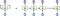h’s stand for output, A stands for the shared neural network, and X’s are inputs. On the right is really just the same network, unrolled through a sequence. image from (http://colah.github.io/posts/2015-08-Understanding-LSTMs/) Olah’s blog

In graph neural networks, the idea of “time step” or “sequence” becomes flexible. An example would be a path in the graph. Producing a sequence of outputs can be thought of as walking the graph, with each step predicting the next node, based on the graph’s structure and a history of nodes visited. Take this example of generating a meaningful sentence: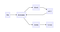A toy example: He dreams that all dreams come true. This is just a toy example. Proper NLP should distinguish between a verb and a noun.

If we start the sentence with “all dreams”, we would like to end with “come true”. Otherwise, we run into an infinite loop: “All dreams that all dreams…”. Thus, it is important for the graph to remember the past path.

Practitioners of traditional RNN have found it hard to preserve information over longer sequences: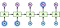The information from X0 and X1 has decaying influence h3, after two propagations. You may imagine the problem gets worse when trying to learn far-reaching dependencies in a sequence (image also from Olah’s blog)

This issue is particularly relevant in graphs because a path may be of arbitrary length, and preserving information over a long “walk” becomes a challenge. This gave birth to improvements such as Long-Short-Term-Memory (LSTM) and Gated-Recurrent-Unit (GRU). A GRU can be thought of as a simplification of LSTM.

Basically, a GRU does not naïvely update its hidden state like traditional RNN. Rather, learnable weights control how much of the previous state to preserve and how much of the current step’s information to absorb. Funneling these weights through an activation function such as Sigmoid drives them towards 0 or 1, which produces a gate-like effect (thus the name). In the previous example of “all dreams”, the gate needs to remember not to “dream” again.

Given GRU’s simplicity compared to LSTM, it scales on massive graphs. Gated Graph Neural Networks (GGNN) are already able to achieve impressive performance on sequential problems [Gated Graph Sequence Neural Networks (Li et al)].

GNN

Although Graph Neural Networks can be understood by similarities with traditional neural networks, a helpful conceptual model unique to GNN is to think of it as message passing between nodes. The message passing consists of 3 steps:

1. Collecting information from neighbors, applying some transformation T on their way
2. Aggregating this information, applying some aggregation function G
3. Updating self-state & weights based on the aggregated representation, with a function U

A simple example:

Suppose we are at node X

T: (int message) → message * w0

Where newInfo is the state of a neighbour. w0 can be some coefficient defined on the edge, such that strongly connected nodes have larger influences on each other’s states.

G: (int[] weightedMessages) → weightMessages.sum()

Where weightedMessages are simply a collection of outputs of T applied on each neighbour of X.

U: (int oldState, int newInfo) → w1*newInfo + (1-w1)*oldState

Where oldState belongs to X and newInfo is the output from G. w1 is a learnable weight from GRU. The output of U will be the new state of the node X.

If we interpret each iteration of T.G.U as a time step, we get a simple Gated Graph Neural Network (GGNN). If we count a node itself as its neighbour and not use GRU by setting w1=1, we get a Graph Convolutional Network (GCN). The number of iterations would correspond to the number of hidden layers.

Note that GNN provides great flexibility in how much of T, G, and U to share. You may use the same weights for T across all edges, edges with a particular label, or different for each edge (but would be expensive on big graphs). The same goes for G and U on nodes.

These 3 steps can be applied iteratively. After one iteration, each node has some information from its direct descendants. After the second iteration, each node has some information from its 2nd level descendants, and so on.

This message-passing model gives the advantage of memory efficiency and explainability — computation is always done locally among a neighborhood — it would not have to perform an operation on the whole network and the human does not have to put the whole network in the head to reason about.

This resembles the process model of an operating system where each process manages a pool of child processes which communicate with each other through message passing.

Use Cases

GNNs can be used quite flexible. In the following examples, Polygon-RNN++ uses GNN only to fine-tune the output, Pixel2Mesh uses a direct mapping between GNN and a graph-oriented problem, and RGBD Segmentation uses graphs to embed structural information to enrich training data.

Polygon-RNN++

This project, by David Acuna, Huan Ling (yes, the speaker), Amlan Kar, and Sanja Fidler, automates the time-consuming image annotation process for computer vision [Efficient Interactive Annotation of Segmentation Datasets with Polygon-RNN++]. This model recognizes a roughly accurate polygon segmentation from an image and takes human adjustments on individual vertices to produce a more accurate segmentation. The tool is open to public here.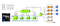A schema for the original Polygon-RNN. GNN is not used here.

In a nutshell, an image first goes through CNN of various sizes and aggregates to a 28x28x128 representation of the image. The representation is then fed into an RNN that predicts one coordinate at a time in a counter-clockwise direction. If the human moves a vertex, all predictions after that vertex will rerun through the RNN with the updated hidden state from the human inputs.

Before GNN fine-tunes the polygon, the resolution of the location prediction of vertices, if you have not yet noticed, is only 28x28. The image is divided into 28 by 28 grids, and no two vertices can live in the same grid. Sometimes, hardware constrains a necessarily small network to be in memory. Adding a GNN layer on the generated polygon enables the network to have an “after-thought” about the prediction.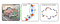The orange dots are from the RNN outputs. The blue dots are initially placed evenly and co-linearly between orange dots. The GNN tries to predict how much and to what direction the blue dots should displace

In particular, the GNN increases the resolution of the polygon by placing a vertex between each pair of adjacent existing vertices and adjusting the magnitude and direction of displacement from its original position based on human input.

Pixel2mesh

This project by Wang et al [Pixel2Mesh: Generating 3D Mesh Models from Single RGB Images] performs better than previous state-of-the-art techniques in generating 3D Mesh representation from 2D images. This is an elegant full-on application of GNN (a mesh model is just a closed 3D surface consisted of many stitched-together small triangles with nodes and edges — A graph!).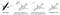3 models try to predict a 3D structure from the 2D image on the left. The first two are based on volumetric description and point cloud description respectively. The third is based on graph description (mesh). There are so many vertices that it looks smooth to the eye. (image from http://bigvid.fudan.edu.cn/pixel2mesh/)

A GNN is constructed directly from the mesh. Computations are directly performed on each node that physically corresponds to a vertex on the mesh. Besides extracting information from the 2D graph, the process consists of iterations of deforming existing vertices and adding new vertices.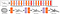The GNN starts from a naive guess — an ellipsoid — and in each iteration, it moves a few vertices around and adds a few more vertices among them (image from the same source as above)

Mesh deformation is achieved by updating a node’s representation with messages from neighbor nodes in a CNN fashion. In equation form: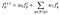One iteration (image from the paper)

f is a representation of vertex position. w0 and w1 are learnable weights shared across all vertices. N(p) gives the neighbors of node p.

Graph unpooling adds new vertices while maintaining each unit surface as a triangle (sounds familiar? See Polygon RNN++ above).

Thanks to the direct mapping between the GNN and the mesh, Wang explains in his paper, defining loss functions become trivial:

• Prefer smooth surfaces
• Discourage vertices from crowding on edges
• Prevent mesh surfaces from cutting into each other

These losses make outputs look more pleasing to the eye than the previous two versions.

A common advantage evident in Pixel2Mesh and Polygon RNN++ is that a graph can represent much more than just the nodes and edges. One can fully describe a polygon just by the borders, and a 3D model just by the surface. The memory footprint grows more slowly than volume-based models when we need to describe a bigger object. This means we can fit a larger model in memory and probably learn higher dimensional features.

RGBD semantic segmentation

Segmenting parts of an image has been a classic computer vision problem. Traditional methods rely only on 2D RGB images. In this project by Qi et al [3D Graph Neural Networks for RGBD Semantic Segmentation], depth information (the distance of each pixel from the camera), collected from devices such as Microsoft Kinetic and dual-camera phones, is included in the form of a graph when performing segmentation. A good example is how to properly segment a mirror, but not getting distracted by the reflection within.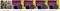Unary CNN © dissected the mirror. RGBD segmentation (e) mostly recovers the mirror as one piece. (image directly from the paper)

How is depth information embedded into a 2D image? One previous attempt is to encode this information as a separate channel (or channels) apart from RGB and uses CNN on this depth information [Gupta et al 2014, Long et al 2015, Eigen et al 2015]. However, using graphs to encode this information delivers better performance. Each node represents a pixel. Pixels close to each other in depth are connected, but those that are close on a 2D grid but distant in depth are not connected with an edge. We include a representation of this graph as inputs to the model.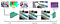From a 2D perspective, both the blue dots and the green dots are close to the red dot. However, with depth information, we can “disconnect” them in the graph. This helps to distinguish background carpet from the foreground bed before any training happens

From the image above, we can see this is almost like cheating because depth information is already able to rule out some pixels as belonging to one object. However, the fact that we can use a graph to encode this information into the neural network helps reduce distractions to the model and can achieve a better accuracy with fewer iterations.

Future Work

GNNs allow both the human and the machine to more accurately model and fit the machine learning task. We can see elements of CNN and RNN being transferred into a graph context. There are still great challenges in this area.

One of the challenges is constructing a graph that accurately describes the data. For RGBD segmentation, there exists flexibility in determining what pixels are considered “close”. The authors used K-Nearest-Neighbours to determine which pixels are connected. However, this is somewhat a compromise. There is no guarantee that each segment has a similar number of points being captured by the device, which would not justify using a fixed K value. In other machine learning problems, researchers have to use a more complex model, such as another neural network (yes, another neural network!) just to create the graph with proper connections, and then train the actual model on the graph. This is essentially similar to the hyperparameter tuning problem, except now there is a whole graph to tune.

There are still a number of problems yet to be applied with GNN, and more challenges yet to be discovered.

The Paper Reading Group is a series of workshops hosted by the University of Toronto Machine Intelligence Student Team (UTMIST). In these workshops, we invite AI researchers to introduce their latest publications to undergraduate students. We hope this helps to ease their learning curve and in clear the mist (hype) around machine learning. While individual researchers decide how technical to go about their papers, our goal is to facilitate connections and foster a community. For more information, you can visit our Facebook page and website.

Works Cited

Xiaojuan Qi, Renjie Liao, Jiaya Jia, Sanja Fidler, Raquel Urtasun. 3D Graph Neural Networks for RGBD Semantic Segmentation. 2017. http://www.cs.toronto.edu/~rjliao/papers/iccv_2017_3DGNN.pdf

Tingwu Wang, Renjie Liao, Jimmy Ba, Sanja Fidler. NerveNet: Learning Structured Policy with Graph Neural Networks. 2017. http://www.cs.toronto.edu/~tingwuwang/nervenet.html

Christopher Olah. Understanding LSTM Networks. 2015. http://colah.github.io/posts/2015-08-Understanding-LSTMs/

Yujia Li, Richard Zemel, Marc Brockschmidt, Daniel Tarlow. GATED GRAPH SEQUENCE NEURAL NETWORKS. 2016. https://arxiv.org/pdf/1511.05493.pdf

David Acuna, Huan Ling, Amlan Kar, Sanja Fidler. Efficient Interactive Annotation of Segmentation Datasets with Polygon-RNN++. 2018. http://www.cs.toronto.edu/polyrnn/

Nanyang Wang, Yinda Zhang, Zhuwen Li, Yanwei Fu, Wei Liu, Yu-Gang Jiang. Pixel2Mesh: Generating 3D Mesh Models from Single RGB Images. 2018. http://bigvid.fudan.edu.cn/pixel2mesh/eccv2018/Pixel2Mesh.pdf